# While Loop in C#

## While Loop in C# with Examples

In this article, I am going to discuss the While Loop in C# Language with Examples. Please read our previous article, where we discussed Loops in C# with Examples. At the end of this article, you will understand what are Looping Statements and their type with examples.

##### While Loop in C# Language:

A loop is nothing but executes a block of instructions or statements repeatedly as long as the loop condition is true. How many times it will repeat means as long as the given condition is true. When the condition fails, it will terminate the loop execution.

A while loop is used for executing a statement repeatedly until a given condition returns false. Here, statements may be a single statement or a block of statements. The loop iterates while the condition is true. If you see the syntax and flow chart parallelly, then you will get more clarity of the while loop.

##### While Loop Syntax in C# Language:

Following is the syntax to use the while loop in C# Language.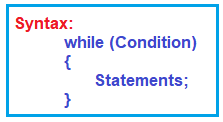While we are working with a while loop first, we need to check the condition, if the condition is true then the control will pass within the body and if the condition is false the control will pass outside the body.

When we are working with an iteration statement after execution of the body control will be passed back to the condition, and until the condition become false. If the condition is not false then we will get an infinite loop.

It is something similar to the if condition, just condition, and statements, but the flow is different from the if condition. How it is different lets us understand through the flow-chart.

##### Flow Chart of While Loop in C# Language:

The following diagram shows the flow chart of the while loop.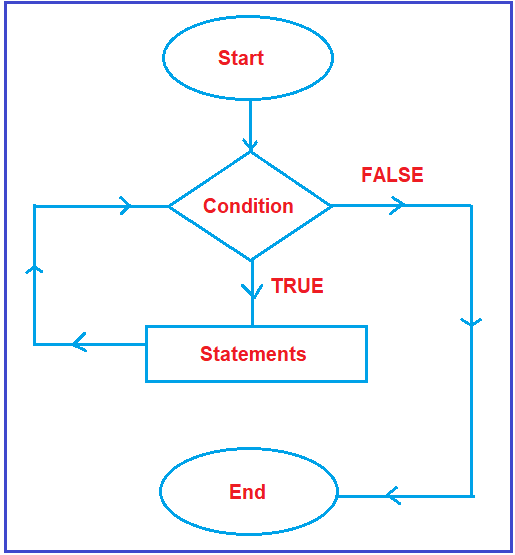The flow chart will start. The start is represented by the oval symbol. Then it will check the condition. As discussed earlier, every condition is having two outputs i.e. true and false. If it is true what will happen and it is false what will happen, we need to check.

Suppose, the condition is true, then what all statements defined inside the block (within the while loop block) will execute. After execution of statements, will it end? NO, it will not end. After execution of statements, once again it will go and check the condition. It will repeat the same process as long as the given condition is true. Suppose, the condition is false, then it will come to end. This is the execution flow of a while loop.

##### Example to understand While loop in C# Language:

In the below example, the variable x is initialized with value 1 and then it has been tested for the condition. If the condition returns true then the statements inside the body of the while loop are executed else control comes out of the loop. The value of is incremented using the ++ operator then it has been tested again for the loop condition.

```using System;
namespace ControlFlowDemo
{
class Program
{
static void Main(string[] args)
{
int x = 1;
while (x <= 5)
{
Console.WriteLine("Value of x:" + x);
x++;
}
}
}
}
```
###### Output: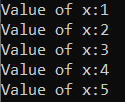##### Example: Print the numbers in the following format up to a given number and that number is entered from the keyboard.

2 4 6 8 …………………….. up to that given number

###### Program:
```using System;
namespace ControlFlowDemo
{
class Program
{
static void Main(string[] args)
{
int i, n;
Console.Write("Enter a Number : ");
i = 2;
while (i <= n)
{
Console.Write(\$"{i} ");
i = i + 2;
}

}
}
}
```
###### Output: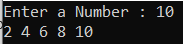##### Example: Enter a number and print the Fibonacci series up to that number using a while loop in C# Language.
```using System;
namespace ControlFlowDemo
{
class Program
{
static void Main(string[] args)
{
int i, n, j, k;
Console.Write("Enter a Number : ");
i = 0;
j = 1;
Console.Write(\$"{i} {j}");

k = i + j;
while (k <= n)
{
Console.Write(\$" {k}");

i = j;
j = k;
k = i + j;
}

}
}
}
```
###### Output: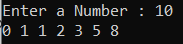##### What is Pre-Checking Process or Entry-Controlled Loop?

The pre-checking process means before the evaluation of the statement block, the conditional part will be executed. When we are working with a while loop always pre-checking process will occur. The loop in which before executing the body of the loop if the condition is tested first then it is called an entry-controlled loop.

While loop is an example of an entry-controlled loop because in the while loop before executing the body first condition is evaluated if the condition is true then the body will be executed otherwise the body will be skipped.

##### Nested While Loop in C# Programming Language:

Writing a while loop inside another while loop is called nested while loop or you can say defining one while loop inside another while loop is called nested while loop. This is the reason why nested loops are also called “loops inside the loop”. There can be any number of loops inside one another with any of the three combinations depending on the complexity of the given problem. In implementation when we need to repeat the loop body itself n number of times then we need to go for nested loops.

##### Nested While Loop Syntax in C# Language:

Following is the syntax to use the nested while loop in the C# language.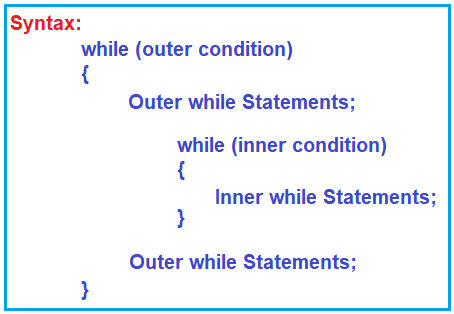Note: In the nested while loop, the number of iterations will be equal to the number of iterations in the outer loop multiplied by the number of iterations in the inner loop. Nested while loops are mostly used for making various pattern programs in C# like number patterns or shape patterns.

##### Execution Flow of Nested While Loop in C# Language:

The outer while loop executes based on the outer condition and the inner while loop executes based on the inner condition. Now let us understand how the nested while loop executes. First, it will check the outer loop condition and if the outer loop condition fails, then it will terminate the loop.

Suppose the outer loop condition is true, then it will come inside, first, it will print the outer loop statements which are there before the inner loop. Then it will check the inner loop condition. If the inner while condition is true, then the control move inside and executes the inner while loop statements. After execution of inner while loop statements, again, it will check the inner while loop condition because it is a loop and as long as the condition is true, it will repeat this process. Once the inner while loop condition fails, then the control moves outside and executes the statements which are present after the inner while loop. Once it executes then, again it will go and check the outer while loop condition. And if it is true, then it will again execute the same process. So, when the loop will terminate means when the outer while loop condition becomes false.

##### Flow Chart of Nested While Loop:

Please have a look at the following diagram, which represents the flow chart of the nested while loop.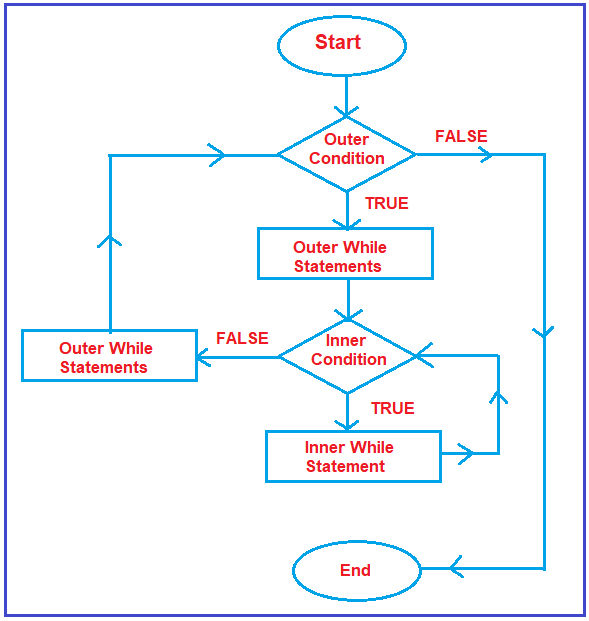The flow will start and first, it will check the outer while loop condition. And if the outer while loop condition failed, then it will come to end. Suppose, the outer loop condition is true, then it will first execute the outer while loop statements if any. After execution of Outer while loop statements, it will check the inner while loop condition. For the inner while loop condition, it will also check for true and false. Suppose, the inner while loop condition is true, then inner while loop statements are executed. After executing the inner while loop statements, again, it will check the inner while loop condition, and this inner loop execution process will repeat as long as the inner while loop condition is true. If the inner while loop condition is false, then the remaining outer loop statements are executed. Once, the outer loop statements are executed, then again, it will come and check the outer while condition. This is the flow of the nested while loop.

##### Example to Print the Following Format using Nested While Loop in C# Language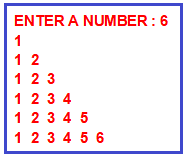###### Program:
```using System;
namespace ControlFlowDemo
{
class Program
{
static void Main(string[] args)
{
Console.Write("ENTER  A NUMBER ");
int i = 1;
while (i <= n)
{
Console.WriteLine();
int j = 1;
while (j <= i)
{
Console.Write(j + " ");
j++;
}
i++;
}

}
}
}
```
##### Example to Print the Following Format using Nested While Loop in C# Language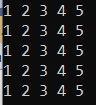###### Program:
```using System;
namespace ControlFlowDemo
{
class Program
{
static void Main(string[] args)
{
int a = 1;
while (a <= 5)
{
int b = 1;
while (b <= 5)
{
Console.Write(b + " ");
b++;
}
Console.WriteLine();
a++;
}

1.